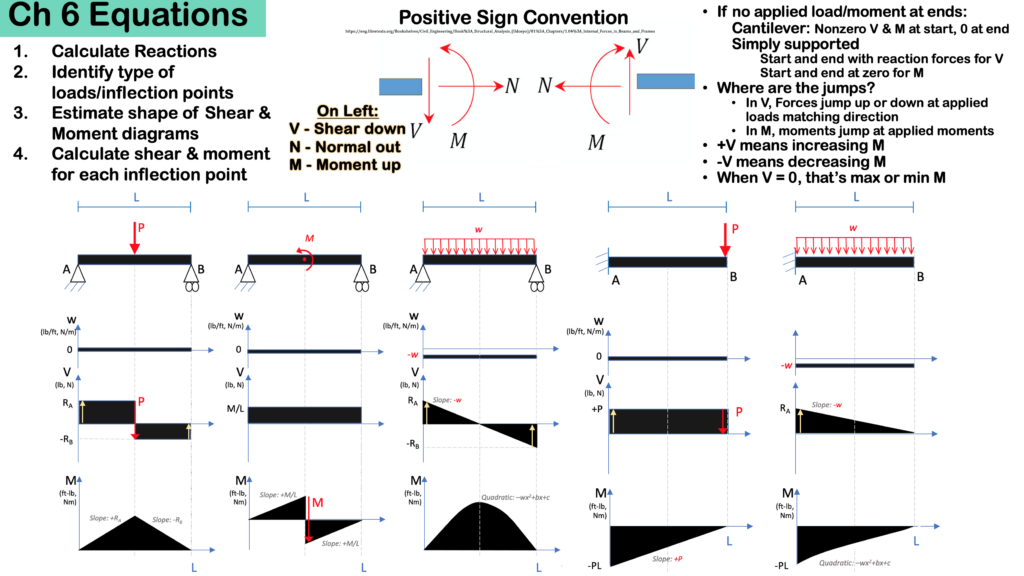# Chapter 6: Internal Forces

In the last chapter we looked at the normal (axial) force running through beams joined into trusses by analyzing either the joints or a whole section of the truss.

In this chapter, we look at what happens along a single beam. We will look at three types of internal forces and moments. Note that when we say ‘internal forces’, we really mean ‘internal forces and moments’. Inside a beam, we will calculate the normal and shear forces as well as the bending moment at any point in the beam.

For this chapter: the shear force and bending moment change throughout the beam because additional transverse forces are applied. However, the normal force usually stays the same, because it’s uncommon to have applied axial forces along the beam.

Here are the sections in this Chapter:

Here are the important equations for this chapter: